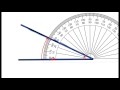#### Year 2##### MathematicsBasic Operations
Divide within 1000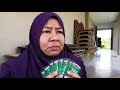#### Year 3##### MathematicsFractions, Decimals and Percentages
Fractions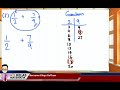Coordinates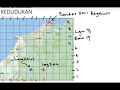#### Year 4##### MathematicsWhole Numbers And Basic Operations
Number value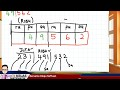Basic operations within 100 000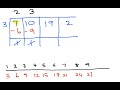Pecahan, Perpuluhan Dan Peratus
Fractions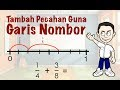FractionsDecimals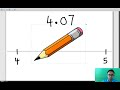Decimals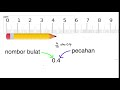Problem solving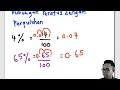Time
12-hour system and 24-hour system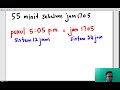Space
Parallel lines and perpendicular lines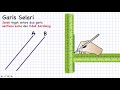Perimeter and area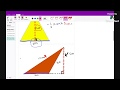#### Year 5##### Mathematics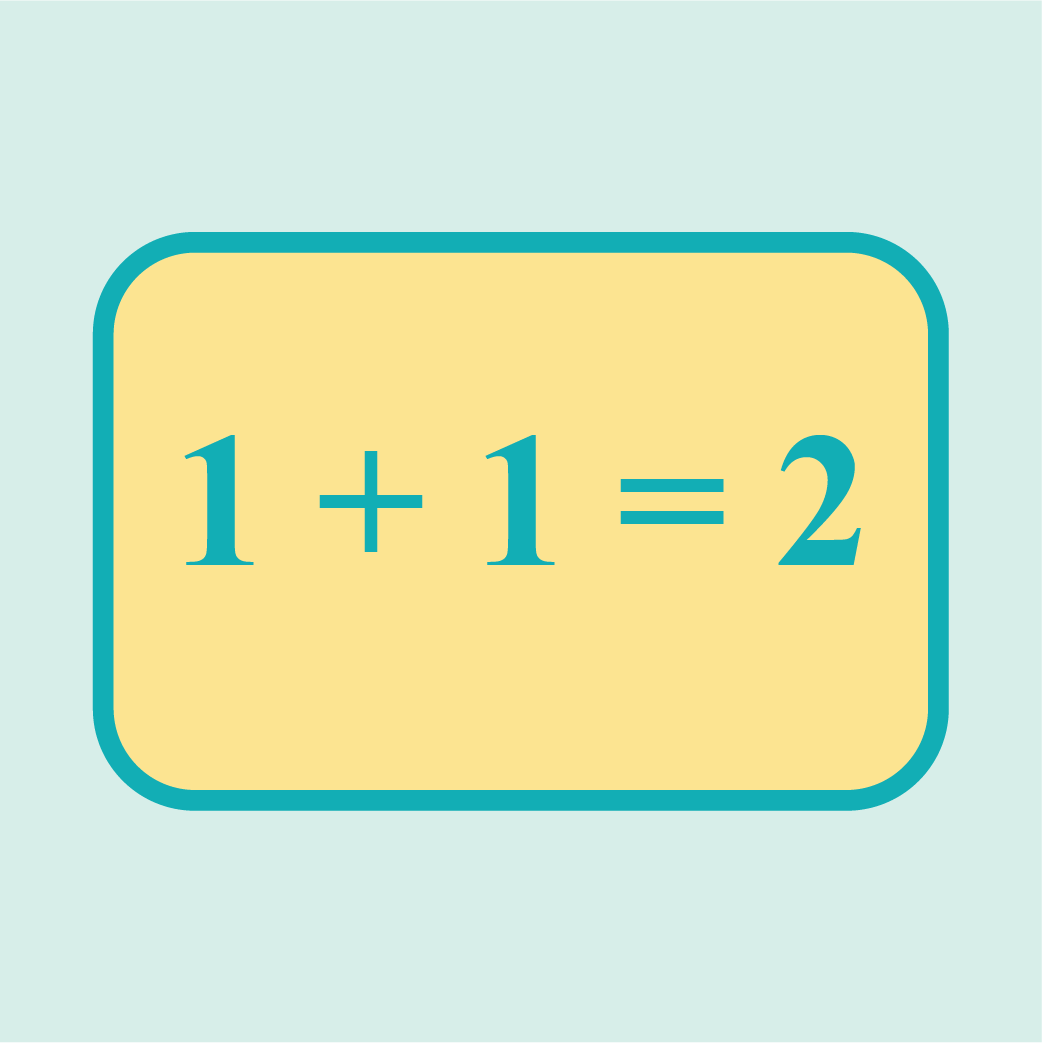Whole Numbers and Basic Operations
Number ValueNumber Value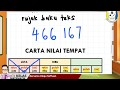Fractions, Decimals and Percentages
Fractions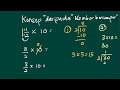FractionsFractionsDecimals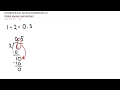Decimals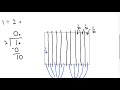Decimals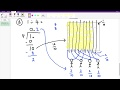Decimals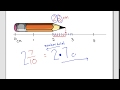Percentages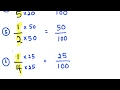Percentages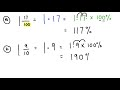Percentages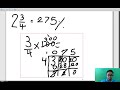Problem solvingMeasurement
Length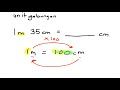Mass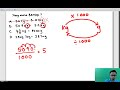Mass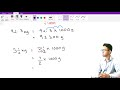Volume of liquid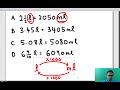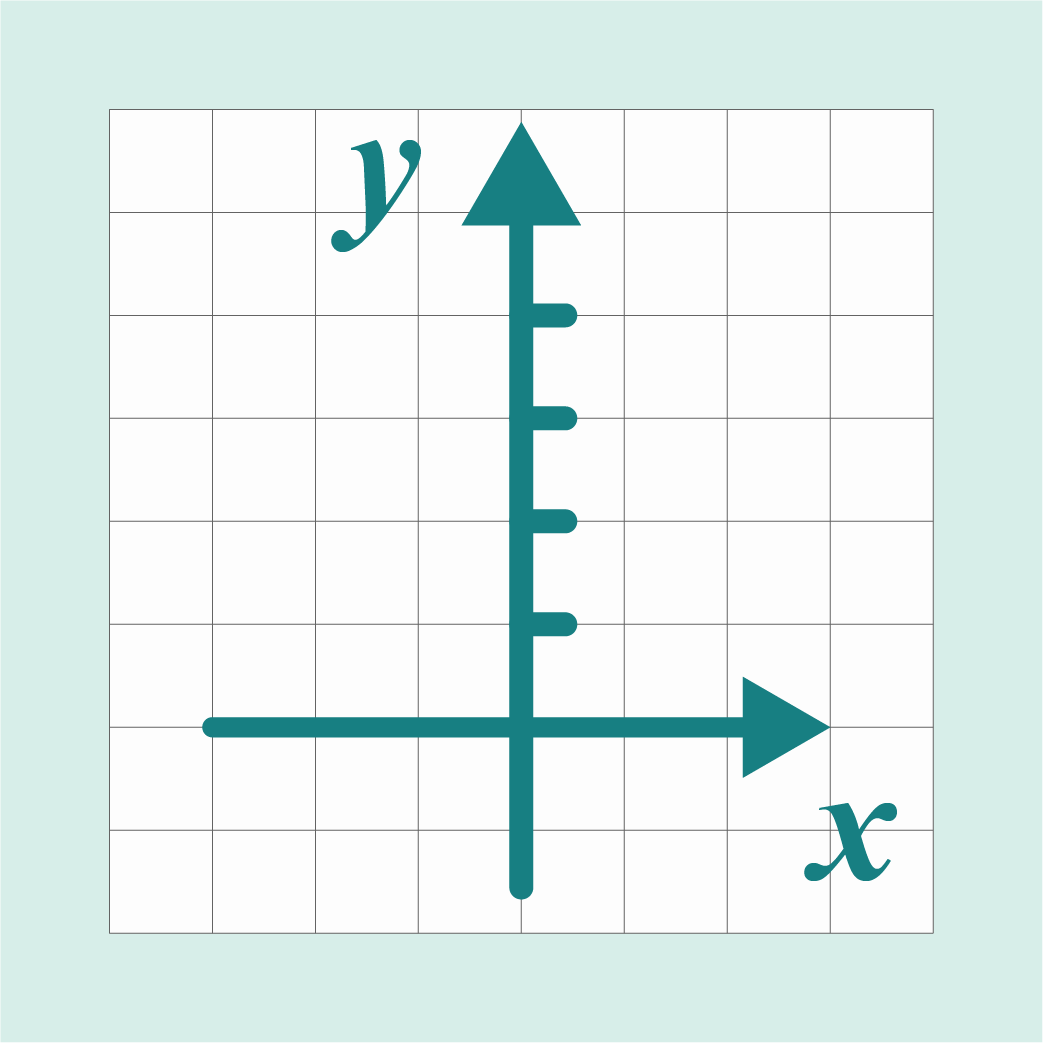Coordinates, Ratio and Proportions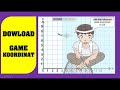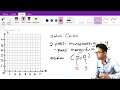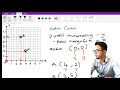Data Handling
Mode, median, mean and range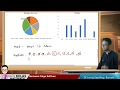Mode, median, mean and range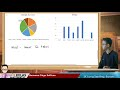#### Year 6##### MathematicsFractions, Decimals And Percentages
Fractions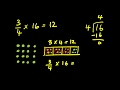Fractions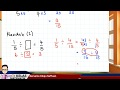Fractions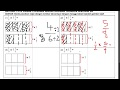Fractions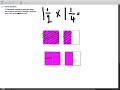Fractions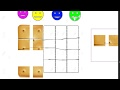Fractions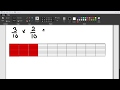Decimals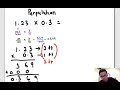Decimals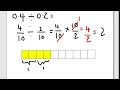Decimals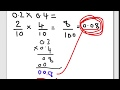Percentages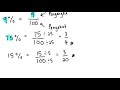Mixed operations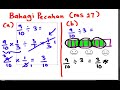Mixed operationsMeasurement
Problem Solving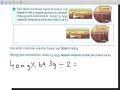Space
Angles